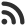Feed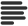Articles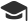Tutorials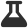Lab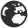CompaniesLeaderboard
 DG Student at BIT Mesra Aug. 18, 2020, 10:18 a.m. ⋅ 2311 views

# CapGemini Pseudo Code Questions with Answer and Explanation (Set 3)

This is set 3 of CapGemini Pseudo Code Question.

Set 1 can be found from here: https://www.hackersfriend.com/articles/capgemini-pseudo-code-questions-with-answer-and-explanation-set-1

Set 2 Can be found from here: https://www.hackersfriend.com/articles/capgemini-pseudo-code-questions-with-answer-and-explanation-set-2

1. What will be the output of following code :

``````#include<stdio.h>
int main()
{
if(sizeof(0))
printf(“Hai”);
else
printf(“Bye”);
return 0;
}``````

A. 2
B. Bye
C. Runtime Error
D. Hai

Ans. D

Explanation : sizeof(0) is 4 hence it is true, Hai will be printed as the message.

2. What will be the output of following code :

``````#include<stdio.h>
int main()
{
if(sizeof(‘\0’))
printf(“inside if block”);
else
printf(“inside else block”);
return 0;
}``````

A. inside if block
B. inside else block
C. Null Pointer Exception
D. None of these

Ans. A

Explanation : A is correct as sizeof(‘\0’) will return 1, hence if condition is true.

3. What will be the output of following code :

``````#include<stdio.h>
int main()
{
int i = 65;
switch(i)
{
case 65:
printf(“Integer 65”);
break;
case ‘A’:
printf(“Char 65”);
break;
default:
printf(“Bye”);
}
return 0;
}``````

A. Integer 65
B. Char 65
C. Bye
D. Error : Duplicate Values

Ans. D

Explanation : D is correct as case ‘A’ and case 65 both are same thing and cases can not have duplicate values.

4. What will be the output of following code :

``````#include<stdio.h>
int main()
{

switch(2/3)
{
case 1:
printf(“case 1 executed “);

case 2:
printf(“case 2 executed “);
break;
default:
printf(“Default block executed”);
}
return 0;
}``````

A. case 1 executed
B. case 2 executed
C. Default block executed
D. Error : Switch statements can not hold

Ans. C

Explanation : C is correct as none of the above cases follow the condition so default block will be executed.

5. What will be the output of following code :

``````#include<stdio.h>
int main()
{
int i = 1;
switch(i)
{
case i:
printf(“case 1 executed”);
break;
case i + 1;
printf(“case 2 executed”);
break;
default:
printf(“default block executed”);
break;
}
return 0;
}``````

A. case 1 executed
B. case 2 executed
C. default block executed
D. Error : i is not usable

Ans. D

Explanation : Above code will produce error: the value of ‘i’ is not usable in a constant expression.

6. What will be the output of following code :

``````#include<stdio.h>
int main(){
while(printf(“%d”, 5) < 4)
printf(“Loop “);
return 0;
}``````

A. Loop Loop Loop Loop Loop
B. Infinite loop
C. 5Loop 5Loop 5Loop 5Loop 5Loop
D. None of these

Ans. B

Explanation : Above code will result in an infinite loop as condition will always be true.

7. What will be the output of following code :

``````#include<stdio.h>
#define NULL 0
int main()
{
while (NULL == 0)
{
printf(“Loop”);
break;
}
return 0;
}``````

A. Loop
B. Null
C. 0
D. Error : Null can not be compared

Ans. A

Explanation : Null is equivalent to 0. And hence, Option A is correct.

8. What will be the output of following code :

``````#include<stdio.h>
int main(){
float ft = 7.5;
while(ft)
{
printf(“Loop”);
ft = ft – .5;
if(ft == 5.0f)
break;
}
return 0;
}``````

A. LoopLoopLoopLoopLoop
B. Loop
C. No output
D. None of these

Ans. A

Explanation : while loop will run for 5 times, i.e for the values 7.5, 7.0, 6.5, 6.0, 5,5. Hence, printing “Loop” for 5 times.

9. What will be the output of following code :

``````#include<stdio.h>
int main()
{
while(!!7)
printf(“Hai”);
return 0;
}``````

A. Hai
B. HaiHai
C. Infinite loop
D. None of these

Ans. C

Explanation : Above code will result in infinite loop.

10. What will be the output of following code :

``````#include<stdio.h>
int main(){
while(!printf(“awesome”));
return 0;
}``````

A. awesome
B. Error
C. Infinite loop
D. None of these

Ans. A

Explanation : Above code will just print “awesome” as the message.

Events

Nov. 28, 2018, 5:30 p.m.

Python from zero to hero

place Delhi

Aug. 13, 2018, 5:30 p.m.

Python from zero to hero

place Bangalore ( HackersFriend office BTM Layout)